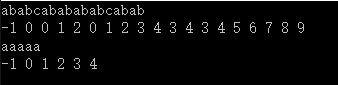# poj_2752 Seek the Name, Seek the Fame（KMP：寻找所有公共前缀后缀）

【题目描述】

The little cat is so famous, that many couples tramp over hill and dale to Byteland, and asked the little cat to give names to their newly-born babies. They seek the name, and at the same time seek the fame. In order to escape from such boring job, the innovative little cat works out an easy but fantastic algorithm:
Step1. Connect the father's name and the mother's name, to a new string S.
Step2. Find a proper prefix-suffix string of S (which is not only the prefix, but also the suffix of S).
Example: Father='ala', Mother='la', we have S = 'ala'+'la' = 'alala'. Potential prefix-suffix strings of S are {'a', 'ala', 'alala'}. Given the string S, could you help the little cat to write a program to calculate the length of possible prefix-suffix strings of S? (He might thank you by giving your baby a name:)

【输入输出】

The input contains a number of test cases. Each test case occupies a single line that contains the string S described above.
Restrictions: Only lowercase letters may appear in the input. 1 <= Length of S <= 400000.

For each test case, output a single line with integer numbers in increasing order, denoting the possible length of the new baby's name.

【输入样例】

ababcababababcabab
aaaaa

【输出样例】

2 4 9 18
1 2 3 4 5

【我的解法】#include <stdio.h>
#include <string.h>

void getNext(char t[], long int next[])
{
int i=0, j=-1, tLen=strlen(t);
next[i]=j;

while (i<tLen)
if (j==-1 || t[i]==t[j])
{
i++;j++;
next[i]=j;
}
else j=next[j];
}

int main()
{
char s;
long int a,next;
while (scanf("%s",s)!=EOF)
{
getNext(s,next);
long int total=-1, sLen=strlen(s), i=sLen, j;
while (next[i]>0) {a[++total]=next[i]; i=next[i];}
for (j=total;j>=0;j--) printf("%ld ",a[j]);
printf("%ld\n",sLen);
}
return 0;
}